# Review — CvT: Introducing Convolutions to Vision Transformers

## Convolutional vision Transformer (CvT)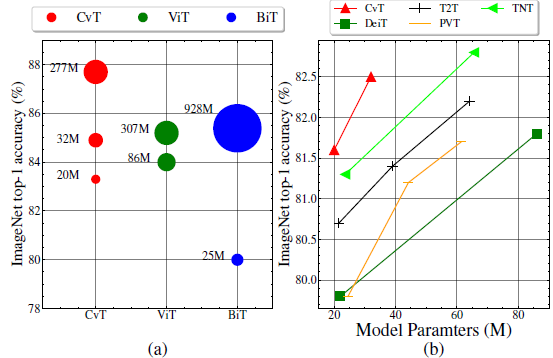Top-1 Accuracy on ImageNet validation compared to other methods with respect to model parameters

CvT: Introducing Convolutions to Vision Transformers
CvT, by McGill University, and Microsoft Cloud + AI
2021 ICCV, Over 200 Citations (Sik-Ho Tsang @ Medium)
Image Classification, Transformer, ViT

• Convolutional vision Transformer (CvT) is proposed through two primary modifications: A hierarchy of Transformers containing a new convolutional token embedding, and a convolutional Transformer block leveraging a convolutional projection.
• These changes introduce desirable properties of convolutional neural networks (CNNs) to the ViT architecture (i.e. shift, scale, and distortion invariance) while maintaining the merits of Transformers (i.e. dynamic attention, global context, and better generalization).

# Outline

1. Convolutional vision Transformer (CvT)
2. Discussions Among ViT Variants
3. SOTA Comparison
4. Ablation Study

# 1. Convolutional vision Transformer (CvT)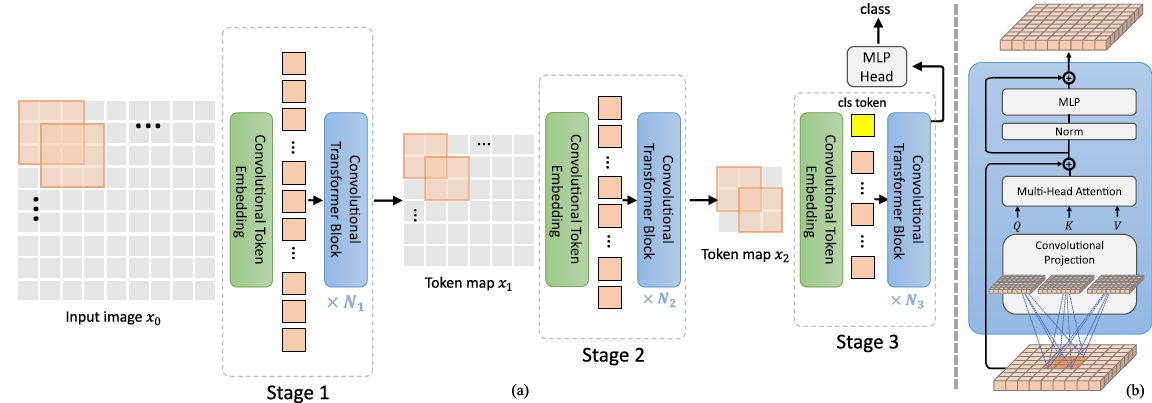The pipeline of the proposed CvT architecture

## 1.1. Overall Architecture

• A multi-stage hierarchy design borrowed from CNNs is employed, where three stages in total are used. Each stage has two parts.
• First, the input image (or 2D reshaped token maps) are subjected to the Convolutional Token Embedding layer (See 1.2), which is implemented as a convolution with overlapping patches with tokens reshaped to the 2D spatial grid as the input (the degree of overlap can be controlled via the stride length).
• An additional layer normalization is applied to the tokens.
• This allows each stage to progressively reduce the number of tokens (i.e. feature resolution) while simultaneously increasing the width of the tokens (i.e. feature dimension), thus achieving spatial downsampling and increased richness of representation, similar to the design of CNNs.
• Different from other prior Transformer-based architectures, the ad-hoc position embedding is not added to the tokens.
• Next, a stack of the proposed Convolutional Transformer Blocks (See 1.3) comprise the remainder of each stage.
• Figure (b) shows the architecture of the Convolutional Transformer Block, where a depth-wise separable convolution operation, referred as Convolutional Projection, is applied for query, key, and value embeddings respectively, instead of the standard position-wise linear projection in ViT.
• Additionally, the classification token is added only in the last stage.
• Finally, an MLP (i.e. fully connected) Head is utilized upon the classification token of the final stage output to predict the class.

## 1.2. Convolutional Token Embedding (Details)

• This convolution operation in CvT aims to model local spatial contexts, from low-level edges to higher order semantic primitives.
• Formally, given a 2D image or a 2D-reshaped output token map from a previous stage xi-1, convolution is applied to obtain the new token map f(xi−1), which has the height and width as follows:
• where s is kernel size, s-o is stride, p is padding.
• f(xi-1) is then flattened into size HiWi×Ci and normalized by layer normalization for input into the subsequent Transformer blocks of stage i.

The Convolutional Token Embedding layer allows us to adjust the token feature dimension and the number of tokens at each stage by varying parameters of the convolution operation. In this manner, in each stage the token sequence length can be progressively decreased, while the token feature dimension is increasing. This gives the tokens the ability to represent increasingly complex visual patterns over increasingly larger spatial footprints, similar to feature layers of CNNs.

## 3.3. Convolutional Projection for Attention (Details)

• The goal of the proposed Convolutional Projection layer is to achieve additional modeling of local spatial context, and to provide efficiency benefits by permitting the undersampling of K and V matrices.
• The original position-wise linear projection for Multi-Head Self-Attention (MHSA) is replaced with depth-wise separable convolutions, forming the convolutional projection.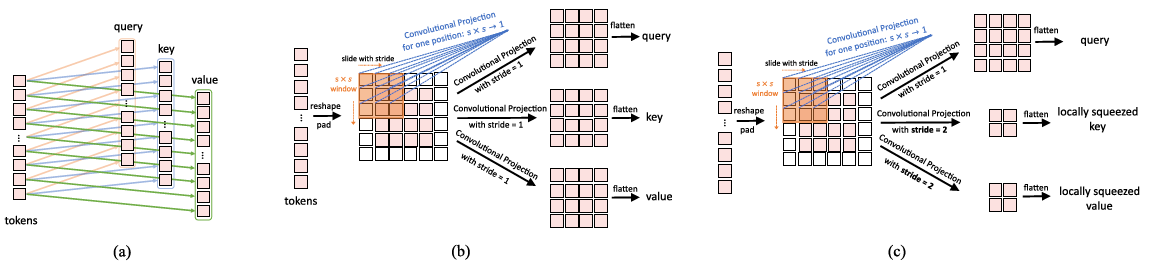(a) Linear projection in ViT (b) Convolutional projection (c) Squeezed convolutional projection
• (a): The original position-wise linear projection used in ViT.
• (b): The proposed s×s Convolutional Projection:
• where xq/k/vi is the token input for Q/K/V matrices.
• Tokens are first reshaped into a 2D token map.
• Next, a Convolutional Projection is implemented using a depth-wise separable convolution layer with kernel size s. Thus, Conv2d is a depth-wise separable convolution implemented by: Depth-wise Conv2d → BatchNorm2d → Point-wise Conv2d.
• Finally, the projected tokens are flattened into 1D for subsequent process.
• (c): The Convolutional Projection, where the key and value projection are subsampled by using a convolution with stride larger than 1, to reduce the computation cost for the MHSA operation.

In this way, the number of tokens for key and value is reduced 4 times, and the computational cost is reduced by 4 times for the later MHSA operation.

## 3.4. CvT Variants

• CvT-13 and CvT-21 are basic models, with 19.98M and 31.54M parameters. CvT-X stands for Convolutional vision Transformer with X Transformer Blocks in total.
• Additionally, a wider model with a larger token dimension for each stage is used, namely CvT-W24 (W stands for Wide), resulting 298.3M parameters.
• A total batch size of 2048 is used for training 300 epochs using 224×224.
• During fine-tuning, a resolution of 384×384 is used with a total batch size of 512.

# 2. Discussions Among ViTVariants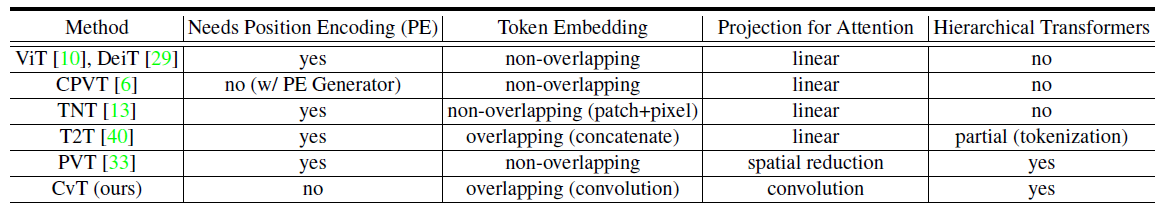Representative works of vision Transformers (ViT)
• The above table tabulates the differences among ViT variants.
• Tokens-to-Token ViT (T2T) implements a progressive tokenization, and then uses a Transformer-based backbone in which the length of tokens is fixed. T2T concatenates neighboring tokens into one new token, leading to increasing the complexity of memory and computation
• By contrast, CvT implements a progressive tokenization by a multi-stage process.
• Both PVT and CvT incorporate pyramid structures from CNNs to the Transformers structure. Compared with PVT, which only spatially subsamples the feature map or key/value matrices in projection, CvT instead employs convolutions with stride to achieve this goal.

# 3. SOTA Comparison

## 3.1. ImageNet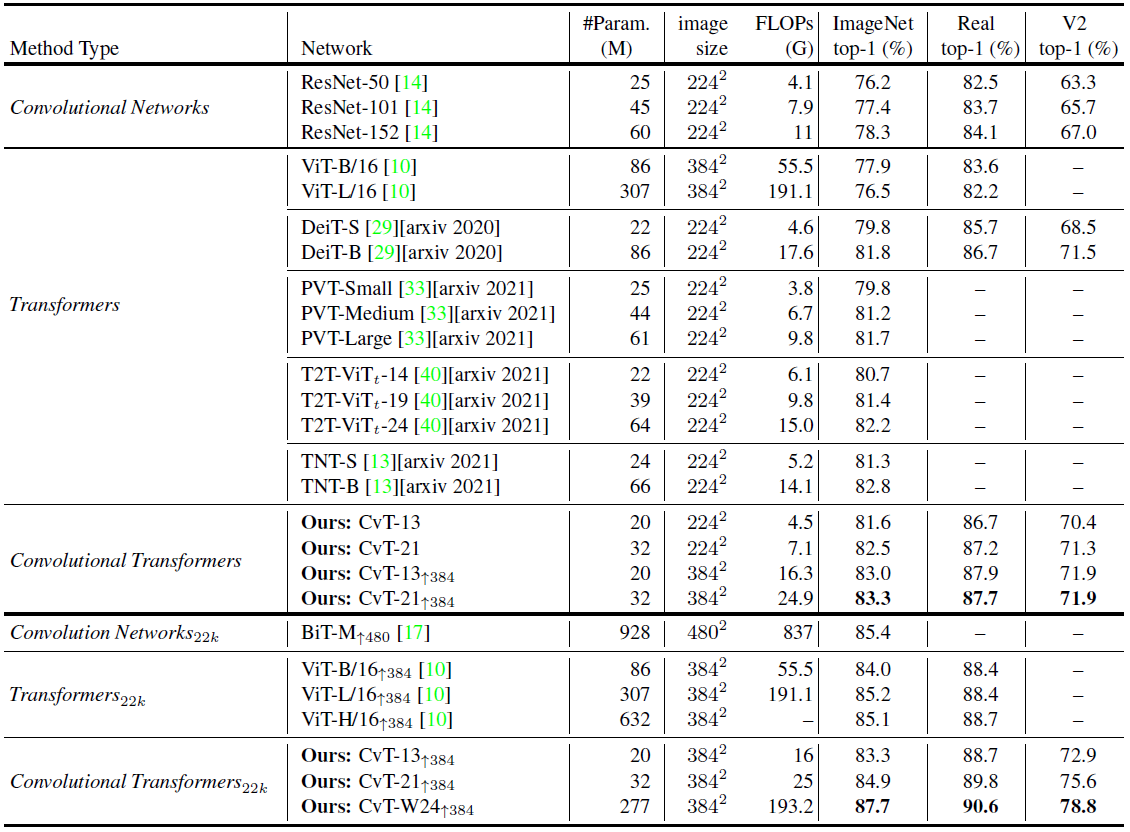Accuracy of manual designed architecture on ImageNet, ImageNet-ReaL and ImageNet-V2
• Compared to Transformer based models, CvT achieves a much higher accuracy with fewer parameters and FLOPs. CvT-21 obtains a 82.5% ImageNet Top-1 accuracy, which is 0.5% higher than DeiT-B with the reduction of 63% parameters and 60% FLOPs.
• When comparing to concurrent works, CvT still shows superior advantages. With fewer parameters, CvT-13 achieves a 81.6% ImageNet Top-1 accuracy, outperforming PVT-Small, T2T-ViTt-14, T2T-S by 1.7%, 0.8%, 0.2% respectively.
• Compared to CNN-based models, CvT further closes the performance gap of Transformer-based models. The smallest model CvT-13 with 20M parameters and 4.5G FLOPs surpasses the large ResNet-152 model by 3.2% on ImageNet Top-1 accuracy, while ResNet-151 has 3 times the parameters of CvT-13.
• Furthermore, when more data are involved, the wide model CvT-W24 pretrained on ImageNet-22k reaches to 87.7% Top-1 Accuracy on ImageNet without extra data (e.g. JFT-300M), surpassing the previous best Transformer based models ViT-L/16 by 2.5% with similar number of model parameters and FLOPs.

## 3.2. Downstream Task Transfer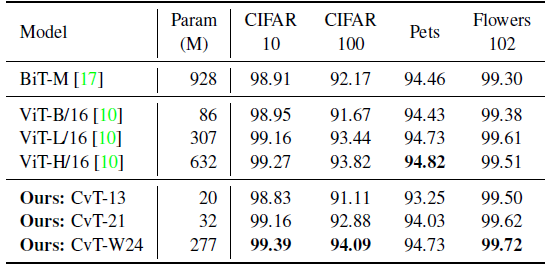Top-1 accuracy on downstream tasks. All the models are pre-trained on ImageNet-22k data
• CvT-W24 model is able to obtain the best performance across all the downstream tasks considered, even when compared to the large BiT-R152×4 model, which has more than 3× the number of parameters as CvT-W24.

# 4. Ablation Study

f: Removing the position embedding does not degrade the performance.

d: Convolutional Token Embedding not only improves the performance, but also helps CvT model spatial relationships without position embedding.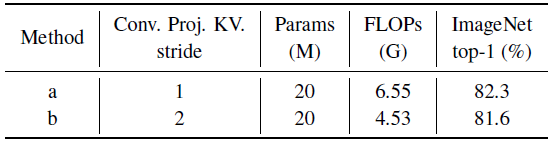Ablations on Convolutional Projection with different strides for key and value projection

b: By using a stride of 2 for key and value projection, a 0.3% drop in ImageNet Top-1 accuracy is observed, but with 30% fewer FLOPs. Thus, this setting is used as default.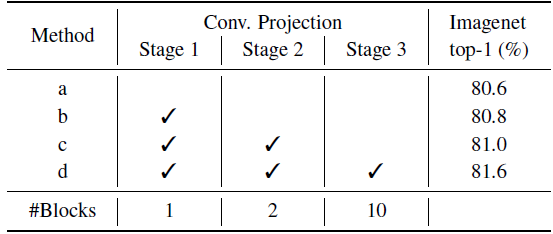Ablations on Convolutional Projection v.s. Position-wise Linear Projection

b-d: Replacing the original Position-wise Linear Projection with the proposed Convolutional Projection improves the Top-1 Accuracy on ImageNet from 80.6% to 81.6%. In addition, performance continually improves as more stages use the design.

## Reference

[2021 ICCV] [CvT]
CvT: Introducing Convolutions to Vision Transformers

## Image Classification

1989 … 2021: [Learned Resizer] [Vision Transformer, ViT] [ResNet Strikes Back] [DeiT] [EfficientNetV2] [MLP-Mixer] [T2T-ViT] [Swin Transformer] [CaiT] [ResMLP] [ResNet-RS] [NFNet] [PVT, PVTv1] [CvT]

--

--